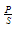Skip to main content

# How to Determine and Use RLC Circuit in Parallel Resonance

### Key Takeaways

• What is an RLC circuit in parallel resonance?

• How are parallel RLC resonant circuits used for PCBA design?

• How to design an RLC circuit in parallel resonant circuits.Blocking unauthorized access.

It is probably safe to say that humanity has, for the most part, transformed into a cyber society. Now that does not mean that we live our lives inside a simulation, like in the movie The Matrix. Instead, I am referring to the fact that computers are intricate and essential parts of our lives that, to a great degree, determine our quality of life. As such, one of our greatest challenges is achieving adequate cybersecurity; thus, being able to provide transparency to the right person(s) when desired while blocking all others.

For PCBA design, data security is also an issue, especially with respect to design data intelligence and when boards will transfer, process, and/or receive medical data. However, a greater problem to engineers and board designers is ensuring that the right signals are passed and all others are rejected. This is typically coupled with designing parallel impedances such that resonance and, thus, maximum power transfer is achieved at the passband center frequency.  Let’s first define what is meant by resonance and then discuss how to best design an RLC circuit in parallel to take advantage of it for optimal signal transmission.What Is Resonance for an RLC Circuit in Parallel?

Generally, resonance can be defined as the point or state at which an externally applied force is at, or very close, to the natural frequency of an object or system. For electrical circuits, this state has a great deal of significance and is the basis for the design of many types of electrical components, devices, and products. For an RLC circuit in parallel, resonance can be defined in three ways, as listed below:

1. ### At unity power factor.

Whenis at maximum.

1. ### At maximum parallel impedance.

Whenis at maximum.

1. ### The frequency at which inductive and capacitive reactances are equal in magnitude.Ideally, the three conditions above all occur at the same resonant frequency. For real circuits and PCBAs, there may be slight discrepancies due to the physical characteristics of components, materials, and traces. Therefore, the latter definition is probably the best criterion to employ for board design and can be used irrespective of any specific circuit usage type.

## Common Usage of Parallel RLC Resonance on Circuit Boards

There is virtually no limit to the different configurations that can be devised that contain some combination of resistance, inductance, and capacitance in parallel. A number of specific combinations serve as models for designing special circuits. Many of these are filters of varying order or type. Common designs are Butterworth, Chebyshev, and Bessel filters. If we limit ourselves to the case where only one resistor, inductor, and capacitor are used, then the design is for a bandpass filter, as shown in the figure below.Example of simple RLC circuit bandpass filter.

Bandpass filters, as their name implies, are designed to pass a specific bandwidth of frequencies. A common feature of parallel RLC filters is the tank circuit where the inductance and capacitance are directly connected in parallel, as shown in the figure above. Tank circuits are the central elements in transmitters, receivers, amplifiers, mixers, oscillators, tuners, and filters.

The LC tank circuit is very important as it defines the resonant frequency of the circuit. At resonance, the reactance of the inductor, XL, and capacitor, XC, are equal in magnitude, and the tank circuit oscillates between storing energy in the magnetic field of the inductor and the electric field of the capacitor. For bandpass filter design, this frequency can be calculated, or set, by choosing a capacitor or inductor which defines the other component using the following equation:Now, the circuit can be tuned as described below.

## Fine Tuning Your Parallel RLC Circuit Filter Design

For a parallel RLC circuit, when the reactive impedances in parallel are equal in magnitude they essentially cancel each other out, as shown in the figure below, and allow for the maximum transfer of power through the resistor.RLC circuit in parallel resonance.

Another important factor for the parallel RLC bandpass filter (and other filters) is the quality factor, Q.

Q=RCL

From this equation, it is obvious that RLC parallel circuits with low resistance also have a low Q factor. The relationship between the values for C and L also affects quality. For example, if L >> C, the passband will be wide; however, if C >> L, the passband will be narrow. Therefore, the choices for component values of R, L, and C can be used to fine-tune the bandpass filter frequency, passband, and amount of power transfer from the source through simulation and analysis.

Filter design can be a tedious back and forth process of picking values, analyzing results, and redesign. However, with Cadence’s PCB Design and Analysis package, this time spent can be greatly reduced. With the Analog/Mixed-Signal Simulation capabilities of PSpice, RLC circuits in parallel tuning can be easily integrated into designs, resulting in a more efficient design process.